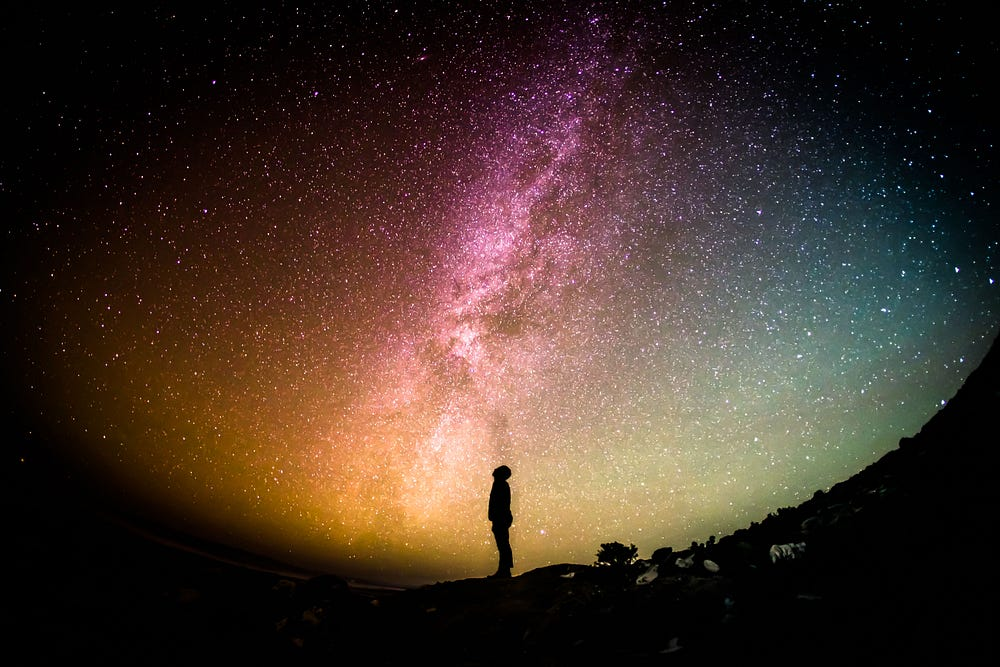# Hacks for Doing Black Magic of Deep Learning

Source: Deep Learning on Medium## Always Overfit

Deep neural networks are known as “black boxes”, where it’s hard to do debugging. And after writing the training scripts, you cant be sure that you don’t have any mistakes in the script or foresee whether your model has enough parameters to learn the transformation that you need.

And that is where the advice about overfiting¹ from Andrej Karpathy is coming.

At the beginning of the training, before feeding all the data to your network, try to overfit it on the one fixed batch, without any augmentation, and with a very small learning rate. If it will not be overfitted, it means, that, either your model doesn’t have enough learning power for transformation that you need, or you have a bug in your code.

Only after successful overfitting, it’s reasonable to start training on the whole data.

Normalization is a strong technique for overcoming vanishing gradients and train network with higher learning rates, without careful parameter initialization. Originally in the paper of S.Ioffe², it’s proposed to normalize features across the batch and turn activations toward the unit Gaussian distribution, to learn one, universal mean and variance, for the all data distribution(test data included). This approach is valid for all classification tasks when you need to predict one(or several in case of multilabel classification) label for the image.
But the picture is different when you are working on image-to-image translation tasks. Here, learning one moving-average and one moving- mean, for the whole dataset may lead to failure. In this case, for each image, as an output of the network, you want to obtain, unique result.
And that’s where instance normalization is coming. In contrast, in instance normalization, the statistics are being computed independently for each image in the batch. And this independence is helping to successfully train networks for such tasks as image super-resolution, neural style transfers, image inpainting and much more.
So be careful and not use the common practice of transfer learning, with the most famous pre-trained networks as ResNet, MobileNet, Inception, in image transformation tasks.

## The Bigger (not always) the Better

It is known, that in the process of training deep neural networks, the bigger batch size is, the faster convergence will be. But also, it is empirically has been shown, that after a certain point, an increase of the batch size can harm the final performance of the model. In the work, N.S. Keskar et. al.³ stated, that it is connected with the fact that in case of the large batches, training tends to converge to sharp minimizers of the training function, and in case of smaller batches, to flat minimizers. As a result, in the first case, there will be high sensitivity from the training function, and little change in data distribution will harm the performance on the test stage.

But further P. Goyal et. al.⁴ in the paper “Accurate, Large Minibatch SGD: Training ImageNet in 1 Hour”, showed that it is possible to train ImageNet with batch size up to 8K, without degradation in performance. As the authors state, optimization difficulty is the main issue with large minibatches, rather than poor generalization (at least on ImageNet). The authors proposed a linear scaling rule for the learning rate, depending on batch size. The rule is following

when the minibatch size is multiplied by k, multiply the learning rate by k.

Small batch size also can be considered as the form of regularization, because in this case, you will have noisy updates, which can help to avoid fast convergence to a local minimum and improve generalization.

## Depthwise Separable Convolution is not Always Your Savior

In recent years, with the increase of performance, the number of parameters in the neural networks increased drastically, and the design of the efficient and less costly neural networks turn out to be an issue of the day.
One of the solutions, proposed by Google as a part of the Tensorflow⁵ framework, is a depthwise separable convolution, which is a modification of the conventional convolutional layer, where you need fewer parameters.

Let us suppose, we have a layer with

fi –input filters

fo -output filters

kh -height of the kernel

kw -width of the kernel

In the case of the convolution, the number of parameters in the layer will be

N = kh * kw * fi * fo

We are convolving each input filter by the number of times of output filters and then summing them up.

And in case of depthwise separable convolution, it will be

N = kh * kw * fi + 1 * 1 * fo

We are convolving each input filter one time, with the kernel (kh, kw), and then, convolving these intermediate filters with the kernel (1, 1), by the number of times of output filters.

Now, let’s have a look at two examples.

## Example 1

Suppose we have following values for the layer

fi = 128

fo = 256

kh = 3

kw = 3

Number of parameters in the convolutional layer will be

3 * 3 * 128 * 256 = 294.912

Number of parameters in depthwise separable convolution will be

3 * 3 * 128 + 1 * 1 * 256 = 99.456

Advantage in case of the depthwise separable convolution is obvious!!!

## Example 2

Now let’s suppose that we have other values for the layer

fi = 128

fo = 256

kh = 1

kw = 1

Number of parameters in the convolutional layer will be

1 * 1* 128 * 256 = 32.768

Number of parameters in depthwise separable convolution will be

1 * 1* 128 + 1 * 1 * 256 = 32.896

So, as we can see, in the second case, instead of having a reduction, we increased the number of parameters.